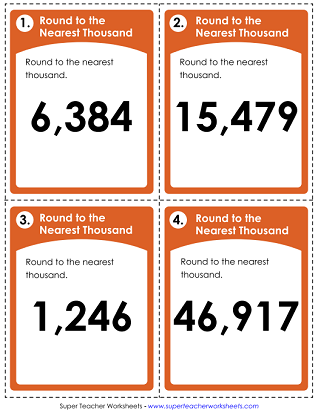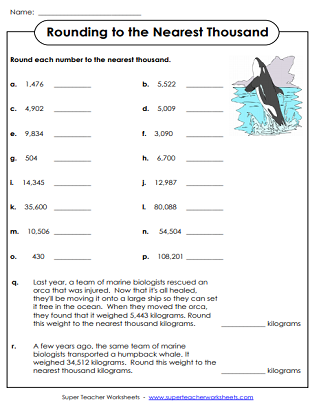# Rounding Numbers to the Nearest Thousand

Here we have a selection of teaching resources that can be used to teach students about rounding numbers to the nearest thousand. Also includes nearest ten, hundred, and thousand mixed.## Nearest Thousand

Part 1: Round the numbers to the nearest thousand. Part 2: Strawberry numbers. Part 3: Add four numbers to the web that round to 8,000. Part 4: True or false.
This page has a set of 3, 4, and 5-digit numbers that students will round to the nearest thousand. There are also two whale-themed word problems.
Kids will begin this activity by using a cypher to decode sets of symbols into numbers. Then they'll round each number.
In this whole-class math game, students will move around the room while rounding numbers to the nearest thousand.
In this printable rounding activity, students will round to the nearest thousand to complete the rounding caterpillar.
This file contains 30 task cards. Round 4-digit numbers to the nearest thousand. Use these cards for classroom games, small group instruction, morning work, or learning centers.
(example: Round 4,512 to the nearest thousand.)
Print out 30 task cards to use for small group instruction, peer tutoring sessions, classroom scavenger hunts, extra practice, or learning centers. This version includes 3, 4, and 5-digit numbers.
(example: Round 34,199 to the nearest thousand.)

## Rounding Ten, Hundred, Thousand (Mixed)

Why did the elephant quit her job at the circus? To find the answer to this funny riddle, students will round numbers to the nearest tens, hundreds, and thousands.
Round numbers to the nearest ten, nearest hundred, and nearest thousand. Then solve the multi-step word problems. This worksheet has an exercise theme.
Compete the rounding table by inserting the missing numbers. Round each number to the nearest ten, hundred, and thousand.
These task cards require students to round to the nearest tens, hundreds, and thousands place.
Decimal Rounding

Practice rounding decimals to the nearest tenth, hundredth, and whole number.

More Rounding Worksheets

Jump to various levels of rounding worksheet, including tens, hundreds, decimals, and fractions.

## Sample Worksheet ImagesMy Account
Site Information